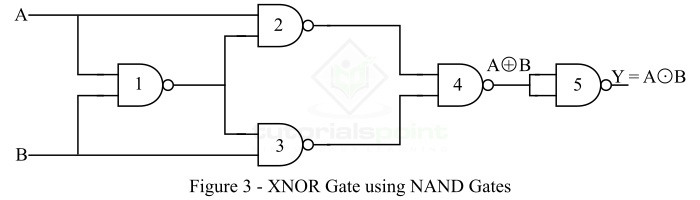# Implementation of XNOR Gate from NAND Gate

As we know, the NAND Gate is a universal logic gate, using which we can implement any other type of logic gate or logical expression. Read this tutorial to find out how you can implement an XNOR gate using only NAND gates. Let's start with a basic overview of XNOR and NAND gates.

## What is an XNOR Gate?

The XNOR (Exclusive-NOR) Gate is a type of derived logic gate. The XNOR gate is a logic gate that has two inputs and one output. The XNOR gate produces a HIGH (Logic 1) output when both of its inputs are equal, i.e. either HIGH (Logic 1) or LOW (Logic 0).

When the inputs of the XNOR gate are different, i.e., one is HIGH (Logic 1) and another is LOW (Logic 0), then the output of the XNOR gate is a LOW (Logic 0) state. The logic symbol of the XNOR gate is shown in Figure-1.Hence, the XNOR gate produces an output HIGH (Logic 1) only when both of its inputs are equal. Thus, the XNOR gate is also known as "equality detector".

The output of the XNOR gate is given by,

$$\mathrm{Y=A\bigodot B=AB + \bar{A}\bar{B}}$$

Where, A and B are the two input variables to the XNOR gate, Y is the output variable of the XNOR gate. The output expression of the XNOR gate is read as Y is equal to A ex-nor B.

### Truth Table of XNOR Gate

The truth table shows the relationship between inputs and output of the XNOR gate. The truth table of an XNOR gate is shown below.

Input

Output

A

B

Y = (AB +A'B')

0

0

1

0

1

0

1

0

0

1

1

1

## What is a NAND Gate?

The NAND Gate is a type of universal logic gate. Where, a universal logic gate is one that can be used to realize any kind logical expression or any other type of logic gate.

A NAND gate is basically a combination of two basic logic gates namely AND gate and NOT gate, i.e.,

$$\mathrm{NAND\:\:Logic = AND\:\:Logic + NOT\:\:Logic}$$

A NAND gate is the type of logic gate whose output is LOW (Logic 0) when all its inputs are high, and its output is HIGH (Logic 1), when any of its inputs is LOW (Logic 0). Therefore, the operation of the NAND gate is opposite that of the AND gate. The logic symbol of a two input NAND gate is shown in Figure-2.### Output Equation of NAND Gate

If A and B are the input variables and Y is the output variable of the NAND gate, then its output is given by,

$$\mathrm{Y=\overline{A\cdot B} = (A\cdot B)'}$$

It is read as "Y is equal to A.B whole bar".

### Truth Table of NAND Gate

The following is the truth table of the NAND gate-

Input

Output

A

B

Y = (A.B)'

0

0

1

0

1

1

1

0

1

1

1

0

Now, let us discuss the implementation of XNOR Gate from NAND Gate.

## Implementation of XNOR Gate from NAND Gate

As discussed above, the NAND gate is a universal logic, using which we can implement any other type of logic gate. The realization of XNOR gate using NAND gates is shown in Figure-3.From the logic circuit diagram of the XNOR gate using NAND gates only, it is clear that we require 5 NAND gates.

Now, let us understand how this NAND logic circuit functions to produce an output equivalent to the XNOR gate-

The output of the first NAND gate is,

$$\mathrm{Y_1=\overline{A B}}$$

The outputs of the secondary and third NAND gates are,

$$\mathrm{Y_2=\overline{A\cdot \overline{AB}}}$$

$$\mathrm{Y_3=\overline{B\cdot \overline{AB}}}$$

These two outputs (Y2 and Y3) are connected to the fourth NAND gate. This NAND gate will produce an output which is,

$$\mathrm{Y=\overline{\overline{A\cdot\overline{AB}}\cdot\overline{B\cdot\overline{AB}}}}$$

$$\mathrm{\Rightarrow Y=A\cdot\overline{AB}+B\cdot\overline{AB}=A(\bar{A}+\bar{B})+B(\bar{A}+\bar{B})}$$

$$\mathrm{\Rightarrow Y= A\bar{A}+A\bar{B}+\bar{A}B+B\bar{B}}$$

$$\mathrm{\therefore Y=A\bar{B}+\bar{A}B=A\bigoplus B}$$

Finally, the output of the fourth NAND gate is input to the fifth NAND gate that functions as an inverter, and produces an output equivalent to the XNOR gate, i.e.,

$$\mathrm{Y=\overline{A\bigoplus B}=A\bigodot B}$$

This is the output of the XNOR gate. Therefore, in this way, we can implement the XNOR gate from NAND gates only.

Updated on: 10-Jan-2023

11K+ Views